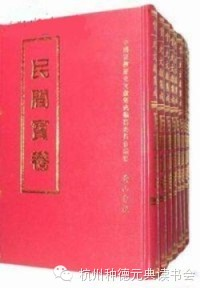财宝卷一卷宝卷一卷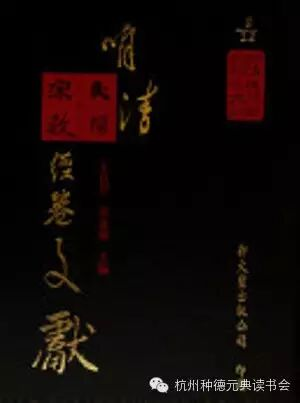《五部六册》雍正七年合校本：

《五部六册》兰风批注本

《五部六册》开心法要版（木刻本）

《五部六册》开心决疑版（抄本）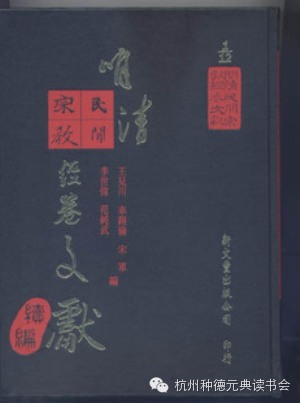```第一册
```
```虎眼禅师传留唱经
```
```普明无为了义丹书宝卷
```
```普明古佛遗留都斗宝赞
```
```普明古佛遗留九祖罗凭收圆宝偈
```
```普明定劫真经
```
```普明古佛遗留考甲文簿
```
```普明古佛遗留五公灵符真宝经
```
```普明如来千佛慈悲利生拔苦真经(卷五)
```
```普明银沙海中取心经
```
```佛说大乘通玄法华真经
```
```皇极九莲儒童临凡宝卷
```
```朝阳遗留三佛脚册唱经偈
```
```朝阳老爷遗留九甲灵文
```
```古佛遗留了言赞
```
```了言赞
```
```弘阳悟道明心经
```
```弘阳妙道玉华随堂真经
```
```销释混元弘阳荐亡本、孤魂调
```
```销释混元弘阳十献、土献、皂经谢圣
```
```混源上严心经
```
```混源下严心经
```
```混源扫心心经
```
```混源悟道心经
```
```蕴空明宝真经(上)
```
```第二册
```
```混源叹世心经
```
```混源破邪心经
```
```销释归家报恩宝卷
```
```圆宗五陀注解玄经
```
```正宗无字注解宝经
```
```显宗下生注解一经
```
```明宗牟尼注解祖经
```
```大乘唐三藏法师留传三煞截鬼经(又名：佛说三煞截鬼经)
```
```指迷引真宝卷
```
```四世行脚觉世宝卷
```
```清静无为妙道真经宝忏
```
```金阙化身玄天上帝宝卷(上)
```
```销释普贤菩萨度华亭县生天宝卷(上)
```
```念佛三昧径路修行西资宝卷
```
```玄天上帝报恩真经(上)(清同治刊本)
```
```玄天上帝报恩真经(民国刊本)
```
```报恩真经忏卷
```
```报恩真经
```
```第三册
```
```参米泥水妙诀金丹宝卷
```
```慧眼皇极静观五色毫光唱经
```
```佛留明经(普明古佛遗留明经)
```
```三圣同降圣寿真经
```
```拔苦真经
```
```三曹普济(卷三至六)
```
```普济真经(中、下)
```
```祖师披示俺姓经
```
```达摩祖师经
```
```大藏血盆经
```
```五圣伏魔真经
```
```新阐五瘟真经全部
```
```三教真经
```
```三教华严经
```
```金刚经
```
```金刚论
```
```根本经
```
```透玲圆觉华严真经
```
```崇正辨异宝鉴
```
```孝道宝卷
```
```第四册
```
```十帝阎罗宝卷(下)(清顺治抄本)
```
```十帝阎罗宝卷(清同治抄本)
```
```梁皇宝卷全集
```
```雪山宝卷
```
```释迦如来雪山宝记
```
```太上老子通化宝卷
```
```罗祖宝卷
```
```何仙姑宝卷
```
```修真宝传
```
```潘公免灾宝卷
```
```圣谕十六条(附：律易解)
```
```新编宣讲大全
```
```宣讲拾遗
```
```玉历至宝钞
```
```第五册
```
```拾诰阴阳醒悟真经灵文
```
```十诰灵文全卷
```
```道脉总源流刊本(梧桐山人辑本)
```
```帖谱
```
```先天道脉科仪——传灯录
```
```玉露金盘
```
```锦囊开展
```
```庆祝表文
```
```表式
```
```皈依文
```
```先天祖回文
```
```杂用表文
```
```祀神礼本解
```
```活世又新
```
```禅门预修
```
```拯世破迷宝诰
```
```苦海金堤
```
```第六册
```
```大乘意讲(又名：皈戒邪恶经)
```
```家谱宝卷
```
```混元家谱卷
```
```佛说家谱宝卷(附：八牛宝赞)
```
```志公经
```
```大圣五公经
```
```五公真经
```
```弥勒尊佛救劫真经
```
```弥勒救劫卷(全部)
```
```弥勒掩姓皇经
```
```弥勒掩姓皇经宝忏
```
```弥勒掩姓皇经礼请
```
```(儒)弥勒三教血盆妙经
```
```好生救劫编
```
```三期度劫经诵本
```
```三教应劫真经
```
```天赐救劫经
```
```王母救劫经
```
```玉皇上帝应验救劫真经
```
```乱世救劫文(拟名)
```
```未来佛普渡经
```
```未来明道真经
```
```报恩经文
```
```四位无字真经
```
```无字真经宝卷
```
```普提换身经忏
```
```三清妙道真经
```
```王母无极妙道三皇(圣)救世皇经
```
```无极先天来明经
```
```光玄古佛指迷经
```
```理数合解
```
```无生老母真经(拟名)
```
```御批瑶池金母救世真经
```
```第七册
```
```关帝忠义觉世真经
```
```关帝忠义觉世真经宝忏
```
```玉律经卷
```
```武圣消劫度人赈济利幽科范
```
```关圣帝君谕免宰杀训
```
```中皇天尊明圣训
```
```武圣帝君血泪救劫文
```
```武圣关壮谬遗迹图志
```
```天后经卷(三种)
```
```天后圣母圣迹图志(两种)
```
```湄洲慈济经
```
```观音大士经文
```
```观音十劝文
```
```高王观世音经缘
```
```司命真君消灾解罪救劫延寿真经
```
```灶王真经
```
```广泽尊王真经
```
```四神志略
```
```第八册
```
```太阳香偈
```
```太阳宝忏
```
```三官宝忏
```
```慈悲血湖宝忏
```
```北斗阐微真经
```
```观音宝忏
```
```文昌宝忏
```
```城隍宝忏
```
```斗母灵签
```
```灵应妙经
```
```钦定梵音大悲灵咒
```
```弥陀忏
```
```香山救父道场
```
```善门日用
```
```醒世要言初集
```
```雷霆鉴
```
```第九册
```
```救生船
```
```劝善宝箴(第二卷)
```
```看破世界
```
```醒迷录
```
```醒迷后录
```
```太上感应篇注案
```
```正道公据
```
```阴阳果报录
```
```第十册
```
```文昌大洞谈经礼请
```
```初颁玉律醒世金丹
```
```石音夫度世成真记
```
```三圣垂训合编
```
```劝世归真
```
```着梦鞭
```
```唤梦醒迷
```
```宝训图书
```
```龙凤图全集
```
```三教同源
```
```第十一册
```
```醒世宝筏
```
```救世宝筏
```
```渡世慈帆
```
```闺阁录(全部)
```
```蓬莱宝训
```
```大成集
```
```八德皈条书本
```
```第十二册
```
```辅正除邪收圆宝训
```
```修真宝筏
```
```十王真经宝忏
```
```临水平妖志
```
```救世宝囊
```
```渡人舟全集
```
```云梦录集
```
```孔圣枕中秘真本
```
```推背图
```
```预言书(三种)
```
```预言书(七种)
```
```玄门捷径
```
```儒宗经卷
```
```孔孟图歌
```
```指南金针(又名：金不换)
```
```吕祖清微三品真经
```
```醒迷要言
```
```(绘图)吕祖八宝救世宝灯
```
```神训南注仙读
```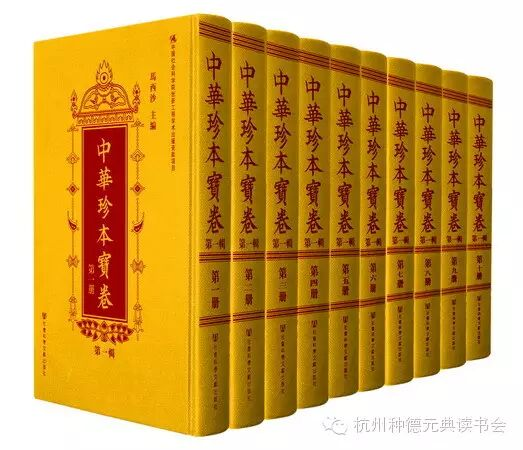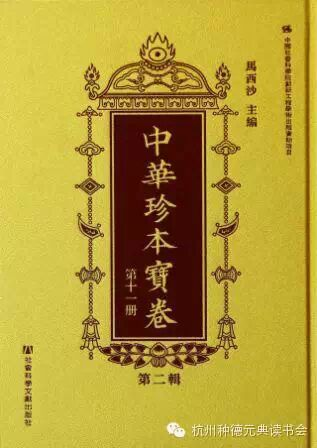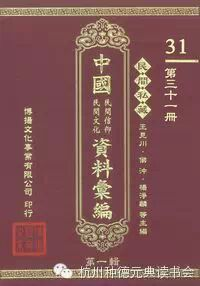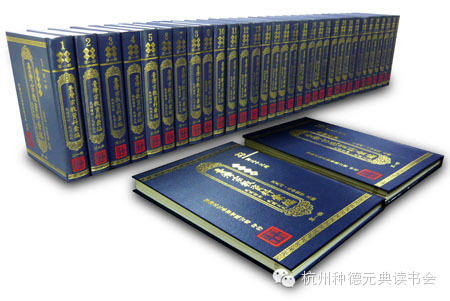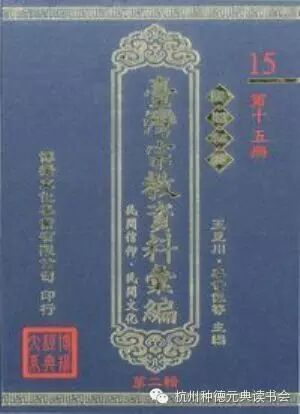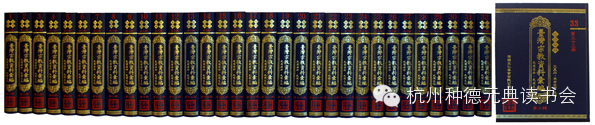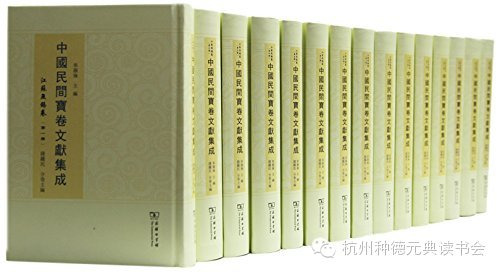Tel. / Fax. 0086-571-28861923 wxzxhsd@126.com hznuwxzhxbgs@163.com